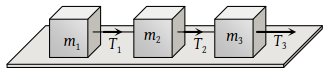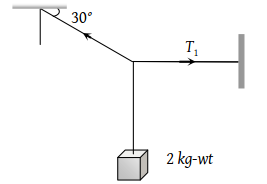You’ve reached the end of your free Videos limit.
#2-Common-Forces-in-Mechanics-Normal-Reaction
(Physics) > Laws of Motion
Unable to watch the video, please try another server
Related Practice Questions :

If rope of lift breaks suddenly, the tension exerted by the surface of lift (a = acceleration of lift)

(1) mg

(2) m(g + a)

(3) m(ga)

(4) 0

High Yielding Test Series + Question Bank - NEET 2020

Difficulty Level:

If two forces of 5 N each are acting along X and Y axes, then the magnitude and direction of resultant is

(1) $5\sqrt{2},\text{\hspace{0.17em}\hspace{0.17em}}\pi /3$

(2) $5\sqrt{2},\text{\hspace{0.17em}\hspace{0.17em}}\pi /4$

(3) $-5\sqrt{2},\text{\hspace{0.17em}\hspace{0.17em}}\pi /3$

(4) $-5\sqrt{2},\text{\hspace{0.17em}\hspace{0.17em}}\pi /4$

High Yielding Test Series + Question Bank - NEET 2020

Difficulty Level:

Three blocks of masses m1, m2 and m3 are connected by massless strings as shown on a frictionless table. They are pulled with a force T3 = 40 N. If m1 = 10 kg, m2 = 6 kg and m3 4 kg, the tension T2 will be(1) 20 N

(2) 40 N

(3) 10 N

(4) 32 N

High Yielding Test Series + Question Bank - NEET 2020

Difficulty Level:

A body of weight 2kg is suspended as shown in the figure. The tension T1 in the horizontal string (in kg wt) is(1) $2/\sqrt{3}$

(2) $\sqrt{3}/2$

(3) $2\sqrt{3}$

(4) 2

High Yielding Test Series + Question Bank - NEET 2020

Difficulty Level:

A false balance has equal arms. An object weigh X when placed in one pan and Y when placed in other pan, then the weight W of the object is equal to

(1) $\sqrt{XY}$

(2) $\frac{X+Y}{2}$

(3) $\frac{{X}^{2}+{Y}^{2}}{2}$

(4) $\frac{2}{\sqrt{{X}^{2}+{Y}^{2}}}$